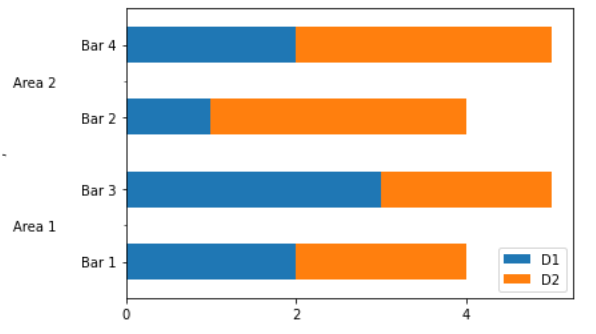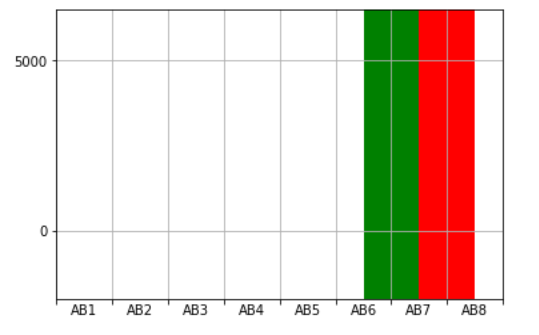# Matplotlib.ticker.AutoMinorLocator Class in Python

Matplotlib is an amazing visualization library in Python for 2D plots of arrays. Matplotlib is a multi-platform data visualization library built on NumPy arrays and designed to work with the broader SciPy stack.

## matplotlib.ticker.AutoMinorLocator

The `matplotlib.ticker.AutoMinorLocator` class is used to find minor tick positions based on the positions of major ticks dynamically. The major ticks need to be evenly spaced along with a linear scale.

Syntax: class matplotlib.ticker.AutoMinorLocator(n=None)

parameter:

• n: it represents the number of subdivisions of the interval between major ticks. If n is omitted or None, it automatically sets to 5 or 4.

Methods of the class:

• tick_values(self, vmin, vmax): Given vmin and vmax it returns the value of the located ticks.

Example 1:

 `import` `pandas as pd ` `import` `matplotlib.pyplot as plt ` `from` `matplotlib ``import` `ticker ` ` `  `data ``=` `[ ` `    ``(``'Area 1'``, ``'Bar 1'``, ``2``, ``2``), ` `    ``(``'Area 2'``, ``'Bar 2'``, ``1``, ``3``), ` `    ``(``'Area 1'``, ``'Bar 3'``, ``3``, ``2``), ` `    ``(``'Area 2'``, ``'Bar 4'``, ``2``, ``3``), ` `] ` ` `  `df ``=` `pd.DataFrame(data, columns ``=``(``'A'``, ``'B'``, ` `                                  ``'D1'``, ``'D2'``)) ` ` `  `df ``=` `df.set_index([``'A'``, ``'B'``]) ` `df.sort_index(inplace ``=` `True``) ` ` `  `# Remove the index names for the plot, ` `# or it'll be used as the axis label ` `df.index.names ``=` `['``', '``'] ` ` `  `ax ``=` `df.plot(kind ``=``'barh'``, stacked ``=` `True``) ` ` `  `minor_locator ``=` `ticker.AutoMinorLocator(``2``) ` ` `  `ax.yaxis.set_minor_locator(minor_locator) ` ` `  `ax.set_yticklabels(df.index.get_level_values(``1``)) ` `ax.set_yticklabels(df.index.get_level_values(``0``).unique(), ` `                   ``minor ``=` `True``) ` ` `  `ax.set_yticks(np.arange(``0.5``, ``len``(df), ``2``),  ` `              ``minor ``=` `True``) ` ` `  `ax.tick_params(axis ``=``'y'``, which ``=``'minor'``,  ` `               ``direction ``=``'out'``, pad ``=` `50``) ` ` `  `plt.show() `

Output:Example 2:

 `from` `pylab ``import` `*`  `import` `matplotlib ` `import` `matplotlib.ticker as ticker ` ` `  ` `  `# Setting minor ticker size to 0,  ` `# globally. ` `matplotlib.rcParams[``'xtick.minor.size'``] ``=` `0` ` `  `# Create a figure with just one  ` `# subplot. ` `fig ``=` `figure() ` `ax ``=` `fig.add_subplot(``111``) ` ` `  `# Set both X and Y limits so that ` `# matplotlib ` `ax.set_xlim(``0``, ``800``) ` ` `  `# Fixes the major ticks to the places ` `# where desired (one every hundred units) ` `ax.xaxis.set_major_locator(ticker.FixedLocator(``range``(``0``, ` `                                                     ``801``,  ` `                                                     ``100``))) ` `ax.xaxis.set_major_formatter(ticker.NullFormatter()) ` ` `  `# Add minor tickers AND labels for them ` `ax.xaxis.set_minor_locator(ticker.AutoMinorLocator(n ``=` `2``)) ` `ax.xaxis.set_minor_formatter(ticker.FixedFormatter([``'AB %d'` `%` `x  ` `                                                    ``for` `x ``in` `range``(``1``, ``9``)])) ` ` `  `ax.set_ylim(``-``2000``, ``6500``, auto ``=` `False``) ` ` `  `# common attributes for the bar plots ` `bcommon ``=` `dict``( ` `    ``height ``=` `[``8500``], ` `    ``bottom ``=` `-``2000``,    ` `    ``width ``=` `100``)       ` ` `  ` `  `bars ``=` `[[``600``, ``'green'``], ` `        ``[``700``, ``'red'``]] ` `for` `left, clr ``in` `bars: ` `    ``bar([left], color ``=` `clr, ``*``*``bcommon) ` ` `  `     `  `show() `

Output:My Personal Notes arrow_drop_upCheck out this Author's contributed articles.

If you like GeeksforGeeks and would like to contribute, you can also write an article using contribute.geeksforgeeks.org or mail your article to contribute@geeksforgeeks.org. See your article appearing on the GeeksforGeeks main page and help other Geeks.

Please Improve this article if you find anything incorrect by clicking on the "Improve Article" button below.

Article Tags :

Be the First to upvote.

Please write to us at contribute@geeksforgeeks.org to report any issue with the above content.